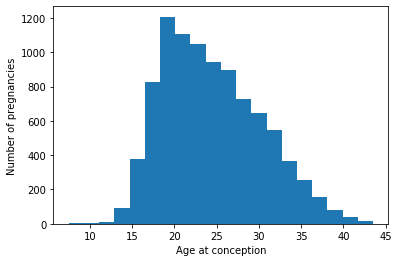DataFrames and Series

Exploring the NSFG data

import pandas as pd
import numpy as np
import matplotlib.pyplot as plt
print(nsfg.shape)

# Display the names of the columns
print(nsfg.columns)

# Select columns birthwgt_oz1: ounces
ounces = nsfg['birthwgt_oz1']

# Print the first 5 elements of ounces
(9358, 10)
Index(['caseid', 'outcome', 'birthwgt_lb1', 'birthwgt_oz1', 'prglngth',
'nbrnaliv', 'agecon', 'agepreg', 'hpagelb', 'wgt2013_2015'],
dtype='object')
0     4.0
1    12.0
2     4.0
3     NaN
4    13.0
Name: birthwgt_oz1, dtype: float64

Clean and Validate

Clean a variable

nsfg['nbrnaliv'].value_counts()
1.0    6379
2.0     100
3.0       5
8.0       1
Name: nbrnaliv, dtype: int64
nsfg['nbrnaliv'].replace(, np.nan, inplace=True)

# Print the values and their frequencies
print(nsfg['nbrnaliv'].value_counts())
1.0    6379
2.0     100
3.0       5
Name: nbrnaliv, dtype: int64

Compute a variable

nsfg['agecon'].describe()
count    9358.000000
mean     2446.330199
std       579.392363
min       750.000000
25%      1983.000000
50%      2366.000000
75%      2850.000000
max      4350.000000
Name: agecon, dtype: float64
nsfg['agepreg'].describe()
count    9109.000000
mean     2494.934570
std       578.099231
min       825.000000
25%      2041.000000
50%      2416.000000
75%      2900.000000
max      4350.000000
Name: agepreg, dtype: float64
agecon = nsfg['agecon'] / 100
agepreg = nsfg['agepreg'] / 100

# Compute the difference
preg_length = agepreg - agecon

# Compute summary statistics
print(preg_length.describe())
count    9109.000000
mean        0.552069
std         0.271479
min         0.000000
25%         0.250000
50%         0.670000
75%         0.750000
max         0.920000
dtype: float64

Filter and visualize

Make a histogram

plt.hist(agecon, bins=20)

# Label the axes
plt.xlabel("Age at conception")
plt.ylabel('Number of pregnancies')
plt.savefig('../images/conception.png')plt.hist(agecon, bins=20, histtype='step')

# Label the axes
plt.xlabel("Age at conception")
plt.ylabel('Number of pregnancies')
Text(0, 0.5, 'Number of pregnancies')Compute birth weight

def resample_rows_weighted(df, column='wgt2013_2015'):
"""Resamples a DataFrame using probabilities proportional to given column.
Args:
df: DataFrame
column: string column name to use as weights
returns:
DataFrame
"""
weights = df[column].copy()
weights /= sum(weights)
indices = np.random.choice(df.index, len(df), replace=True, p=weights)
sample = df.loc[indices]
return sample
nsfg = resample_rows_weighted(nsfg, 'wgt2013_2015')

# Clean the weight variables
pounds = nsfg['birthwgt_lb1'].replace([98, 99], np.nan)
ounces = nsfg['birthwgt_oz1'].replace([98, 99], np.nan)

# Compute total birth weight
birth_weight = pounds + ounces/16
full_term = nsfg['prglngth'] >= 37

# Select the weights of full-term babies
full_term_weight = birth_weight[full_term]

# Compute the mean weight of full-term babies
print(full_term_weight.mean())
7.409452970741339

Filter

full_term = nsfg['prglngth'] >= 37

# Filter single birth
single = nsfg['nbrnaliv'] == 1

# Compute birth weight for single full-term babies
single_full_term_weight = birth_weight[single & full_term]
print('Single full-term mean:', single_full_term_weight.mean())

# Compute birth weight for multiple full-term babies
mult_full_term_weight = birth_weight[~single & full_term]
print('Multiple full-term mean:', mult_full_term_weight.mean())
Single full-term mean: 7.421643309222423
Multiple full-term mean: 5.765243902439025